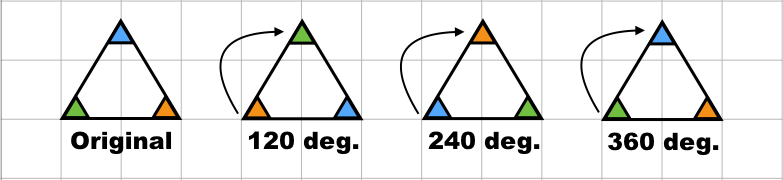# 4.5: Symmetry

$$\newcommand{\vecs}{\overset { \rightharpoonup} {\mathbf{#1}} }$$ $$\newcommand{\vecd}{\overset{-\!-\!\rightharpoonup}{\vphantom{a}\smash {#1}}}$$$$\newcommand{\id}{\mathrm{id}}$$ $$\newcommand{\Span}{\mathrm{span}}$$ $$\newcommand{\kernel}{\mathrm{null}\,}$$ $$\newcommand{\range}{\mathrm{range}\,}$$ $$\newcommand{\RealPart}{\mathrm{Re}}$$ $$\newcommand{\ImaginaryPart}{\mathrm{Im}}$$ $$\newcommand{\Argument}{\mathrm{Arg}}$$ $$\newcommand{\norm}{\| #1 \|}$$ $$\newcommand{\inner}{\langle #1, #2 \rangle}$$ $$\newcommand{\Span}{\mathrm{span}}$$ $$\newcommand{\id}{\mathrm{id}}$$ $$\newcommand{\Span}{\mathrm{span}}$$ $$\newcommand{\kernel}{\mathrm{null}\,}$$ $$\newcommand{\range}{\mathrm{range}\,}$$ $$\newcommand{\RealPart}{\mathrm{Re}}$$ $$\newcommand{\ImaginaryPart}{\mathrm{Im}}$$ $$\newcommand{\Argument}{\mathrm{Arg}}$$ $$\newcommand{\norm}{\| #1 \|}$$ $$\newcommand{\inner}{\langle #1, #2 \rangle}$$ $$\newcommand{\Span}{\mathrm{span}}$$$$\newcommand{\AA}{\unicode[.8,0]{x212B}}$$

In 2D geometry, a figure is symmetrical if an operation can be done to it that leaves the figure occupying an identical physical space. This can be accomplished in two ways.

## Line symmetry

Line symmetry occurs when a line may be passed through an object such that both halves of the object perfectly mirror each other.

Example $$\PageIndex{1}$$:

Consider the triangle below. An equilateral triangle has three instances of line symmetry: one from each vertex to the midpoint of the opposite side.## Rotational Symmetry

Rotational symmetry occurs when a shape may be rotated to occupy the same space as the original.

Example $$\PageIndex{2}$$:

Let's take the same triangle again. An equilateral triangle has three degrees of rotational symmetry: at 120, 240, and 360 degrees. Once we have determined rotational symmetry to 360 degrees, we can stop, as the pattern will repeat itself after that.## Symmetry in Art

Symmetry in art is used as a way to emphasize beauty and order. The human brain finds symmetry to be attractive and beautiful. For this reason, artists attempt to emphasize (at certain times and using certain methods) the symmetry of their subject or surroundings.

Triskelions - an example of rotational symmetry.This page titled 4.5: Symmetry is shared under a CC BY-NC-SA license and was authored, remixed, and/or curated by Pamini Thangarajah.